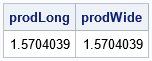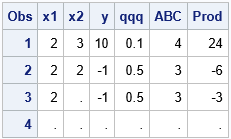A SAS programmer noticed that there is not a built-in function in the SAS DATA step that computes the product for each row across a specified set of variables. There are built-in functions for various statistics such as the SUM, MAX, MIN, MEAN, and MEDIAN functions. But no DATA step function for the product.

This article discusses various ways to compute products in SAS. The article shows how to use PROC FCMP to implement a PRODUCT function that can be called from the DATA step.

### Wide and long data

If you have numbers in a rectangular data set, you might want to compute a product in either of two directions: down a column or across a row. The SAS programmer was interested in computing across a row, but, for completeness, I will demonstrate both cases.

Let's generate some sample data on which to compute the product. For fun, the data I will use is the first 1,000 terms of the Wallis product, which is an infinite sequence of numbers whose product converges to π/2 ≈ 1.5707963. The product of the first 1,000 terms of the Wallis sequence is 1.5704039. The following two DATA steps generate the Wallis terms. The WallisLong data set contains one variable and 1,000 rows. The WallisWide data set contains 1,000 variables and one row. The names of the variables are term1, term2, ..., term1000.

```/* Create sample data. See https://blogs.sas.com/content/iml/2021/03/10/pi-and-products.html */ %let N = 1000; data WallisLong(drop=n); do n = 1 to &N; term = (2*n/(2*n-1)) * (2*n/(2*n+1)); output; end; run;   data WallisWide(drop=n); array term[&N]; do n = 1 to &N; term[n] = (2*n/(2*n-1)) * (2*n/(2*n+1)); end; output; run;```

### The PROD function in SAS/IML

Before computing the products in the SAS DATA step, I want to mention that SAS/IML software supports a built-in function that can compute the product of the nonmissing elements of its arguments. You can use PROD function in PROC IML to read the data and compute the products, as follows:

```/* the easy way: SAS/IML */ proc iml; use WallisLong; read all var "term" into TermLong; close; use WallisWide; read all var _NUM_ into TermWide; close;   prodLong = prod(TermLong); /* compute product of 1000 x 1 column */ prodWide = prod(TermWide); /* compute product of 1 x 1000 row */ print prodLong prodWide; QUIT;```### Using a DO loop and an array to compute a product

You can write a program to compute a product in the DATA step. If there are no missing values in the data, the program is straightforward. However, it is best to write programs that can handle missing values in the data. To detect missing values, you can use the MISSING function. The following program computes the product down the rows and excludes any missing values. If all values are missing, then the product is a missing value.

```/* DATA step method to compute the product down the rows */ data _null_; retain Prod 1 n 0; /* n = number of nonmissing */ set WallisLong end=EOF; if ^missing(term) then do; n + 1; Prod = Prod * term; end; if EOF then do; if n=0 then Prod=.; put n= / prod=; end; run;```
```n=1000 Prod=1.570403873```

The DATA step program to compute the product across multiple columns is similar. It uses an ARRAY statement to loop over the variables term1-term1000. Again, the product omits missing values.

```/* DATA step method to compute the product across columns */ data _null_; array term[&N]; /* implicit assumption: Variables are named term1-term1000 */ set WallisWide; Prod = 1; n = 0; /* n = number of nonmissing */ do i = 1 to dim(term); if ^missing(term[i]) then do; n + 1; Prod = Prod * term[i]; end; end; if n=0 then Prod = .; put n= / Prod=; run;```

For this example, the variables are named term1-term1000, so it is easy to read the variables into the array. For more complicated examples, you can read about ways to specify a list of variable names in SAS.

### Define the PRODUCT function in PROC FCMP

Although the program in the previous section shows how to compute the product for each row across multiple variables, it is more useful to encapsulate that logic into a function that you can call from the DATA step. You can use PROC FCMP to define a function that you can call from the DATA step. The following call to PROC FCMP defines the PRODUCT function. The input argument is an array:

```proc fcmp outlib=work.funcs.MathFuncs; function Product(x[*]); prod = 1; n=0; /* n = number of nonmissing */ do i = 1 to dim(x); if ^missing(x[i]) then do; n + 1; prod = prod * x[i]; end; end; if n=0 then prod = .; return( prod ); endsub; quit;   /* call user-defined PRODUCT function for an array */ options cmplib=(work.funcs); /* tell DATA step where to search for user-defined functions */   data _null_; array vars[&N] term1-term1000; /* array of all variables that will be in the product */ set WallisWide; Prod = Product(vars); /* send that array to the user-defined function */ put Prod=; run;```
`Prod=1.570403873`

### Handling missing values

Whenever you write a user-defined function, you should practice defensive programming and assume that someday your function will be called on data that have missing values. For completeness, the following program creates data that contains missing values. The next program shows an alternate way to define the array if the variables do not have a common prefix and a numerical suffix:

```data Have; input x1 x2 y qqq ABC; datalines; 2 3 10 0.1 4 2 2 -1 0.5 3 2 . -1 0.5 3 . . . . . ;   data Want; set Have; array vars ABC qqq x2 x1 y; /* list variable names in any order */ Prod = Product(vars); run;   proc print; run;```### Summary

This article shows how to implement a user-defined function that computes the product of multiple variables for each row in a data set. The function is defined by using PROC FCMP and is callable from any SAS DATA step. Although you might not need to use this particular function, it provides a good example of how to create a user-defined function in SAS. It also shows how to pass an array to an FCMP function.

Share